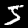# Neural Networks From Scratch with a twist

There have been lots of "Neuronal Network from Scratch" articles lately. So you may ask, why for the love of god would you write yet another.

While being part of the Julia Community and their machine learning efforts for quite a while, I think I can add a unique perspective on the matter.

While most articles that implement DNNs from scratch only work for toy examples, I will show how to build them while maintaining production ready performance. This works out pretty well thanks to Julia's unique strengths in this area - so you may also read this article to learn about some of Julia's main advantages for user friendly high performance programming. Furthermore, I will explain basic DNN concepts in a more clutter free way, like back propagation and automatic differentiation.

These three perspectives, achieving state of the art performance quickly, learning about Julia and explaining nasty details in an easy way were enough motivation to write yet another article :)

So, for the readers that are less into the topic, let's start with a very general explanation of what a DNN is.

## High Level view of a DNN

In its very core, any DNN is very simple. It's basically a black box, that contains a huge tunable function with millions of parameters. Training that function will result in the parameters to be fine tuned to some problem, to mold the function into returning the right answers:

Inside the tunable functions, we usually have lots of layers, made up by smaller functions. Those functions can be any function that has parameters we can tune and an input/output. In reality, they contain mostly a few functions that have been proofen to be effective:

• softmax (exp.(x) ./ sum(exp.(x)))
• dense (W * x .+ b)
• relu (max(zero(x), x))
• convolution

## Tuning a.k.a Back-Propagation

#TODO, actually, I feel like I could come up with an even better example to visualize the basic work horse of a DNN

utilities.jl
include(utilities.jl)

function next_position(position, angle)
position .+ (sin(angle), cos(angle))
end

# Our tunable function ... or chain of flexible links
function predict(chain, input)
output = next_position(input,  chain) # Layer 1
output = next_position(output, chain) # Layer 2
output = next_position(output, chain) # Layer 3
output = next_position(output, chain) # Layer 4
return output
end

function loss(chain, input, target)
sum((predict(chain, input) .- target) .^ 2)
end

chain = [(rand() * pi) for i in 1:4]

input, target = (0.0, 0.0), (3.0, 3.0)
weights, s = visualize(chain, input, target)
s
using Zygote
# first index, to get gradient of first argument
end
for i in 1:100
# get gradient of loss function
# update weights with our loss gradients
# this updates the weights in the direction of smaller loss
chain .-= 0.01 .* angle∇
# update visualization
weights[] = chain
sleep(0.01)
end;

## From Scratch

TODO: describe all the things

using Colors, ImageShow
import Zygote, Flux

glorot_uniform(dims...) = (rand(Float32, dims...) .- 0.5f0) .* sqrt(24.0f0/sum(dims))

struct Dense{M <: AbstractMatrix, V <: AbstractVector, F <: Function}
W::M
b::V
func::F
end

function Dense(in, out, func = identity)
Dense(glorot_uniform(out, in), zeros(Float32, out), func)
end

function (a::Dense)(x::AbstractArray)
a.func.(a.W * x .+ a.b)
end

softmax(xs) = exp.(xs) ./ sum(exp.(xs))

relu(x::Real) = max(zero(x), x)

function crossentropy(ŷ::AbstractVecOrMat, y::AbstractVecOrMat; weight = 1)
-sum(y .* log.(ŷ) .* weight) * 1 // size(y, 2)
end
crossentropy (generic function with 1 method)
0.3s
Julia
function forward(network, input)
result = input
for layer in network
result = layer(result)
end
return result
end
loss(network, x, y) = crossentropy(forward(network, x), y)
# first index, to get gradient of first argument
end

for field in propertynames(b)
end
end
for (alayer, blayer) in zip(a, b)
end
end
"""
We use standard Gradient descent for nothing as Optimizer
"""
a .-= 0.1 .* b
end

function train!(network, X, Y, optimizer = nothing, epochs = 100)
for epoch in 1:epochs
@show epoch
end
end

function test(n)
img = X[1:28^2, n:n]
predict = Tuple(argmax(forward(network, img))) - 1
@show predict
save("/results/test.png", Gray.(reshape(img, (28, 28))))
return nothing
end
test (generic function with 1 method)
network = (
Dense(28^2, 32, relu),
Dense(32, 10),
softmax
)
imgs = Flux.Data.MNIST.images()
labels = Flux.Data.MNIST.labels()
Y = Flux.onehotbatch(labels, 0:9)
X = Float32.(hcat(float.(reshape.(imgs, :))...))
train!(network, X, Y)
using FileIO
test(1)